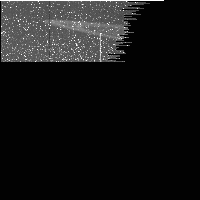# Galileo SSI Image C0552599400

## PDS Label Information:

``````
CCSD3ZF0000100000001NJPL3IF0PDS200000001 = SFDU_LABEL

/* File Format and Length */
RECORD_TYPE = FIXED_LENGTH
RECORD_BYTES = 1000
FILE_RECORDS = 810

/* Pointers to Data Objects */
^TELEMETRY_TABLE = ("9400R.IMG",3)
^IMAGE = ("9400R.IMG",11)
^LINE_PREFIX_TABLE = ("9400R.IMG",11)

/* Description/Catalog Keywords */
DATA_SET_ID = "GO-J/JSA-SSI-2-REDR-V1.0"
SPACECRAFT_NAME = "GALILEO ORBITER"
INSTRUMENT_NAME = "SOLID STATE IMAGING SYSTEM"

/* Time tags and observation descriptors */
SPACECRAFT_CLOCK_CNT_PARTITION = 1
SPACECRAFT_CLOCK_START_COUNT = "05525994.00"
IMAGE_TIME = 2000-05-21T12:29:46.388Z
IMAGE_ID = "28R0001"
ORBIT_NUMBER = 28
OBSERVATION_ID = "28RSRINGS_01"
TARGET_NAME = "J RINGS"
NTV_TIME_FROM_CLOSEST_APPROACH = "000T07:37:42Z"
NTV_SAT_TIME_FROM_CLOSEST_APRH = "000T07:37:42Z"
NOTE = "NOT APPLICABLE"

/* Camera and spacecraft state parameters */
FILTER_NAME = "CLEAR"
FILTER_NUMBER = 0
EXPOSURE_DURATION =   795.83
GAIN_MODE_ID = "10K"
FRAME_DURATION =    8.667
LIGHT_FLOOD_STATE_FLAG = "ON"
EXPOSURE_TYPE = "NORMAL"
BLEMISH_PROTECTION_FLAG = "OFF"
INVERTED_CLOCK_STATE_FLAG = "NOT INVERTED"
ON_CHIP_MOSAIC_FLAG = "N"
COMPRESSION_TYPE = "BARC RATE CONTROL"
ENTROPY =    4.642
TELEMETRY_FORMAT_ID = "IM4"
MEAN_TRUNCATED_BITS =    0.000
MEAN_TRUNCATED_SAMPLES =  291.399
OBSTRUCTION_ID = "NOT POSSIBLE"
INSTRUMENT_MODE_ID = "N/A"

/* Viewing Geometry */
/* Note: These viewing geometry parameters are best estimates */
/* at the time this picture label was generated. */
POSITIVE_LONGITUDE_DIRECTION = WEST

/* Spacecraft Geometry */
TARGET_CENTER_DISTANCE = 6.386123e+05
CENTRAL_BODY_DISTANCE = 6.386123e+05
SUB_SPACECRAFT_LATITUDE =    0.446
SUB_SPACECRAFT_LONGITUDE =   67.826
SUB_SPACECRAFT_LINE = 3124.403
SUB_SPACECRAFT_LINE_SAMPLE = 20269.443
SUB_SPACECRAFT_AZIMUTH =  247.887

/* Camera and Lighting Geometry                        */
/* Resolution of HORIZONTAL_PIXEL_SCALE,               */
/* VERTICAL_PIXEL_SCALE, and SLANT_DISTANCE            */
/* is calculated from the light source values in       */
/* INTERCEPT_POINT_LATITUDE, INTERCEPT_POINT_LONGITUDE,*/
/* INTERCEPT_POINT_LINE and INTERCEPT_POINT_LINE_SAMPLE keywords  */
/* If the target is a Ring keyword RING_RADIUS         */
/* is substituted for INTERCEPT_POINT_LATITUDE         */
/* If the TARGET_NAME = J RINGS, viewing geometry was */
/* calculated using Jupiter as the target. */
TWIST_ANGLE =  103.969
CONE_ANGLE =  161.395
RIGHT_ASCENSION =   25.521
DECLINATION =   12.073
NORTH_AZIMUTH =   98.496
SMEAR_AZIMUTH = "UNK"
SMEAR_MAGNITUDE = "UNK"
HORIZONTAL_PIXEL_SCALE = 7.244560e+03
VERTICAL_PIXEL_SCALE = 6.943180e+03
SLANT_DISTANCE = 4.970330e+05
SOLAR_DISTANCE = 7.463550e+08
SUB_SOLAR_LATITUDE =    3.125
SUB_SOLAR_LONGITUDE =   58.310
SUB_SOLAR_AZIMUTH =  189.316
INCIDENCE_ANGLE =   86.875
EMISSION_ANGLE =   89.427
PHASE_ANGLE =   20.976
LOCAL_HOUR_ANGLE = UNK
INTERCEPT_POINT_LONGITUDE =   35.000
INTERCEPT_POINT_LINE = 7.7
INTERCEPT_POINT_LINE_SAMPLE = 783.4

/* Processing parameters */
MEAN_REFLECTANCE = "N/A"
REFLECTANCE_SCALING_FACTOR = "N/A"
UNEVEN_BIT_WEIGHT_CORR_FLAG = "N/A"
DARK_CURRENT_FILE_NAME = "N/A"
SLOPE_FILE_NAME = "N/A"
BLEMISH_FILE_NAME = "N/A"
SHUTTER_OFFSET_FILE_NAME = "N/A"
DATA_TYPE = LSB_UNSIGNED_INTEGER
SOURCE_PRODUCT_ID = {"S001024A.BSP","S001024A.BSP","N/A","CKG28ABA.PLT","SSI_S
NIP.G28AGD_FIXED_PICNOS_ACTID"}
PROCESSING_HISTORY_TEXT = "VICAR programs run: SSIMERGE,CATLABEL,BADLABELS."
PRODUCT_TYPE = "REDR"

/* BARC Encoding */
CUT_OUT_WINDOW = {1,1,248,800}

/* VICAR IMAGE HEADER Object */
INTERCHANGE_FORMAT = BINARY
BYTES = 2000
RECORDS = 2
^DESCRIPTION = "VICAR2.TXT"
END_OBJECT

/* Table Object (for telemetry table) */
OBJECT = TELEMETRY_TABLE
INTERCHANGE_FORMAT = BINARY
ROWS = 1
COLUMNS = 86
ROW_BYTES = 1800
^STRUCTURE = "RTLMTAB.FMT"
END_OBJECT

INTERCHANGE_FORMAT = BINARY
BYTES = 6000
RECORDS = 6
END_OBJECT

/* Image Object */
OBJECT = IMAGE
LINES = 800
LINE_SAMPLES = 800
SAMPLE_BITS = 8
SAMPLE_TYPE = UNSIGNED_INTEGER
INVALID_CONSTANT = "N/A"
LINE_PREFIX_BYTES = 200
^LINE_PREFIX_STRUCTURE = "RLINEPRX.FMT"
END_OBJECT
END
``````